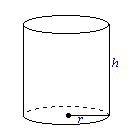# Calculating the Volume of a Cylinder

To calculate the volume of a cylinder, you need to know either the diameter or radius and the height. Once you have those measurements, all you have to do is to plug in the needed information into the cylinder volume formula. Why do I say you either have to know the diameter or radius? If you are given the radius, you are good to continue. If you are given the diameter, you can find the radius easily by taking the number and dividing by two. The cylinder volume formula isV = π r2h.

V is the volume, r is the radius of the circle base, and h is the height.

Why does π rlook familiar in the volume formula you might ask. That is the formula for the area of the circle which is the base. To find the volume, you need to take the base and multiply by the height. That is why the formula is what it is.

Lets say the radius is 5 inches and the height is 10 inches.

V = π (5)2(10)  = 250π in3

That is all there is to it. Now you will know how much will fit into every cylinder you have.

*Please note that if you only know the diameter, you will have to divide by two to find the radius.

Why is this useful information to know? It comes especially in handy for construction workers/engineers. Pipes come in all different sizes, and the way to figure out the best size for a particular job is to know how much volume is there. The bigger the volume, the more liquid/gas gets through in a faster amount of time. There are many other uses for knowing the volume of a cylinder though. Please comment your thoughts below!